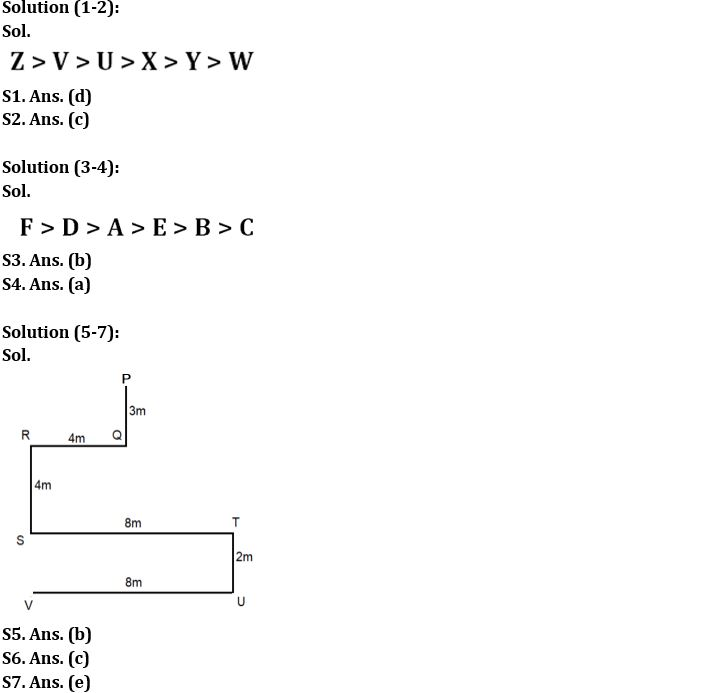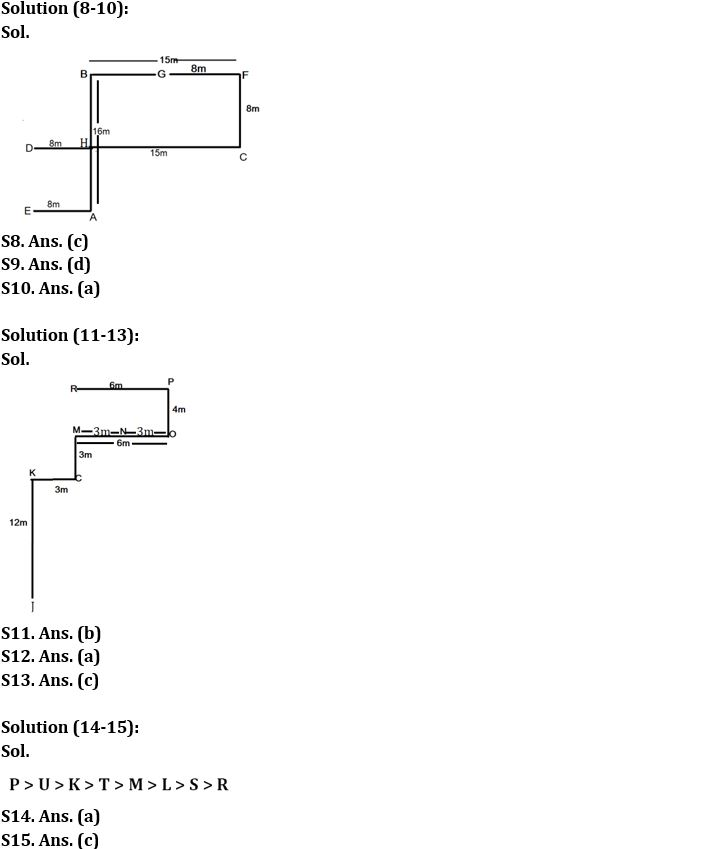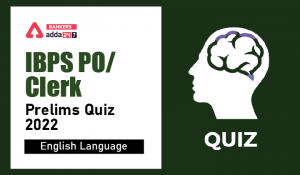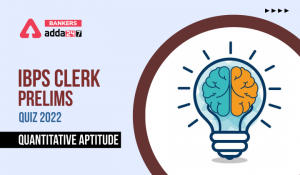Latest Banking jobs   »

# Reasoning Ability Quiz For IBPS Clerk Prelims 2022- 29th July

Directions (1-2): Answer the questions based on the information given below.
Six persons U, V, W, X, Y and Z have different weights. X is heavier than at least two persons. V is lighter than only one person. Z is heavier than Y who is heavier than W. U is heavier than X but not the heaviest.

Q1. How many persons are lighter than X?
(a) Three
(b) Four
(c) Five
(d) Two
(e) None of these

Q2. Who among the following person is 3rd heaviest?
(a) X
(b) Y
(c) U
(d) W
(e) Z

Directions (3-4): Answer the questions based on the information given below.
Six children (A, B, C, D, E and F) have different number of vehicles. E has more vehicles than only two persons. A has more vehicles than B and C but less than F. D has more vehicles than A. B does not have lowest number of vehicles. D does not have highest number of vehicles.

Q3. Which child has the 2nd highest number of vehicles?
(a) F
(b) D
(c) A
(d) E
(e) None of these

Q4. How many children are there in between D and C?
(a) Three
(b) Four
(c) One
(d) Two
(e) None of these

Directions (5-7): Answer the questions based on the information given below.
Deepak starts walking from point P in south direction. After walking 3m he reaches at point Q. He turns right from point Q and walks for 4m till point R. He then turns left from point R and walks for 4m till point S and then turns left from point S and walks for 8m till point T. He then turns right from point T and walk for 2m till point U and then turns right from point U, walks for 8m till point V and stops.

Q5. In which direction is point V with respect to point T?
(a) South
(b) South-west
(c) North
(d) North-east
(e) North-west

Q6. What is the shortest distance between point R and point V?
(a) 10m
(b) 14m
(c) 6m
(d) 8m
(e) 12m

Q7. In which direction is point R with respect to point U?
(a) South
(b) South-west
(c) North
(d) North-east
(e) North-west

Directions (8-10): Answer the questions based on the information given below.
Eight points A, B, C, D, E, F, G and H are drawn on paper. G is 8m west of F which is 15m east of B. B is 16m north of A which is 8m east of E. H is 15m west of C which is 8m south of F. H is 8m east of D.

Q8. What is the direction of F with respect to E?
(a) North-west
(b) South-west
(c) North-east
(d) South-east
(e) None of the above

Q9. What is the shortest distance between D and E?
(a) 5m
(b) 6m
(c) 7m
(d) 8m
(e) None of the above

Q10. What is the direction of G with respect to C?
(a) North -west
(b) South -west
(c) North -east
(d) South-east
(e) None of the above

Directions (11-13): Answer the questions based on the information given below.
Nine friends J, K, L, M, N, O, P, Q & R sit in a park in a certain arrangement. J sits 12m south of K. O sits 6m east of M. C sits 3m east of K. M sits 3m north of C. N sits exactly in the middle of M and O. P sits 4m north of O. R sits 6m west of P.

Q11. What is the direction of J with respect to R?
(a) North-west
(b) South- west
(c) North- east
(d) South- east
(e) None of the above

Q12. What is the shortest distance between N and P?
(a) 5m
(b) 6m
(c) 7m
(d) 8m
(e) None of the above

Q13. What is the direction of P with respect to K?
(a) North-west
(b) South- west
(c) North- east
(d) South- east
(e) None of the above

Directions (14-15): Answer the questions based on the information given below.
Eight persons have different amount of money with them. K has more money than T who has more money than M and L. L has more money than S but lesser money than M. Only P has more money than U. R has less money than U. M has more money than S who has second least amount of money.

Q14. Who has 3rd least amount of money?
(a) L
(b) R
(c) T
(d) M
(e) None of the above

Q15. How many persons have less money than K?
(a) Three
(b) Four
(c) Five
(d) Two
(e) None of these

Solutions#### Congratulations!Incorrect details? Fill the form again here

•English Quizzes For IBPS Clerk/PO Prelim...
•English Quizzes For IBPS Clerk/PO Prelim...
•Quantitative Aptitude Quiz For IBPS Cler...
•Quantitative Aptitude Quiz For IBPS Cler...
•Reasoning Ability Quiz For IBPS Clerk/PO...
•Quantitative Aptitude Quiz For IBPS Cler...